# Free Convection in a Caterpillar Shaped Triangular Enclosure Filled with Different Nanofluids

Free Convection in a Caterpillar Shaped Triangular Enclosure Filled with Different Nanofluids
Tripura Institute of Technology, Agartala, Tripura 799009, India

National Institute of Technology, Agartala, Tripura 799046, India

Corresponding Author Email:
triveni_mikky@yahoo.com
Page:
398-406
|
DOI:
https://doi.org/10.18280/ijht.370204
7 January 2018
|
Accepted:
17 July 2018
|
Published:
30 June 2019
| Citation

OPEN ACCESS

Abstract:

A numerical work is conducted for the free convection in a right triangular cavity filled with water-based nanofluids. The bottom wall is in a caterpillar wavy shape which is assumed as a hot wall whereas the rest walls of the enclosure are considered as a cold wall. Governing equations of the problem are discretized through the finite volume method. The present study is undertaken to appraise the effect of the constrained parameters i.e. various types of nanofluids (TiO2, CuO, and Al2O3), volume fractions of thenanoparticles and Rayleigh number (Ra=105-107). The higher augmentation in the rate of heat transfer is observed for the Al2O3-water-based nanofluid for each Rayleigh number.

Keywords:

natural convection, triangular enclosure, nanofluid, Rayleigh number

1. Introduction

The exploration of the heat transfer through free convection in enclosures with irregular shapes play a prominent role in industrial applications and hence it has become a subject of interest in these present days. However, enclosures like triangular shaped have received a paramount attention in many practical appliances, for example, thermal environment of buildings, solar energy systems, cooling of electronics equipment, geophysics, nuclear power plant, heat exchanger and many other applications involving heat transfer due to its geometry [1-4]. Morsil and Sabeur-Bendehina  conducted numerical study for natural convection in a wavy square enclosure. It was reported that the Nusselt number was decreased with the undulations. Bhavnani and Bergles  reported the natural convection in the sinusoidal wavy surfaces. It was mentioned that the heat transfer rate was increased by 15 % at amplitude to wavelength ratio of 0.3. Rahman et al.  analyzed the effect of buoyancy ratio (Br) and Lewis number (Le) on the heat and mass transfer. On the same cavity, Rahman et al.  studied the heat transfer in a corrugated base surface triangular enclosure. Results revealed that both heat and mass transfer was enhanced with the increase of the wavelength and Rayleigh number.

The conventional fluids such as water, air, oil, and ethylene glycol are used as heat conveyance fluids, but it is quite challenging to use these fluids for momentous improvements in the heat transfer because of their inherently poor heat carrying capabilities. On the other hand, emerging applications like microelectronics, micro-electro-mechanical system (MEMS), and nanotechnology offered devices in miniaturized form exaggerates the thermal loads of the system. Hence, to overcome these challenges, the role of heat transport fluids becomes intrinsic in the management of enhanced thermal loads. An innovative technique where small solid nanoparticles which are suspended in the base or pure fluid generally called nanofluid was initially introduced by Choi  to reform the drawback of the base fluids. It was assessed that the increment of the nanoparticles concentrations in base fluid enhances the thermal conductivity of the nanofluid and hence, substantially, the rate of heat transfer. Afterward, plenty of models have been developed to calculate the thermal conductivity of the nanofluid by various researchers  so that the heat transfer rate can be enhanced.

Aside from the individual study of the Irregular shapes and nanofluids, some researchers have studied the natural convection in an enclosure with these two parameters together. Esmaeilpour and Abdollahzadeh  examined the natural convection in an enclosure filled with nanofluid for different patterns of wavy walls. Shermet et al.  studied the natural convection in a wavy open porous cavity filled with nanofluid. Abu-Nada et al.  numerically investigated the natural convection in Al2O3-water nanofluid filled wavy enclosure. It was found that the adiabatic wavy wall influenced the heat transfer. Sheikholeslami  used CuO-water nanofluid in the sinusoidal cavity to investigate the natural convection. Cho et al.  numerically analyzed the natural convection in a wavy enclosure filled with water-based nanofluid. Ghasemi and Aminossadati  numerically solved a natural convection problem in a right triangular enclosure filled with CuO-water nanofluid. The Brownian motion effects of nanoparticles were considered in the study. They stated that the Brownian motion significantly participated in the thermal performance of the enclosure filled with nanofluid. Later on, Sheikhzadeh et al.  also emphasized that the Maxwell-Granet model is not much effective as it does not consider the rownian motion of the nanoparticles and urged to use the model which consider the Brownian motion. Ghasemi and Aminossadati  again conducted a numerical study on mixed convection in right triangular cavity filled with nanofluid. Rahman et al.  studied the natural convection in a corrugated base wall triangular shape solar collector filled with different nanofluids. The heat transfer rate was augmented by 24.28 % with 0 to 10 % increment of the volume fraction for Cu-nanofluid at Gr=106.

The existing literature unveiled that both surface geometry and nanofluids play an essential role in the enhancement of the heat transfer rate. However, the amalgamation of these two i.e. irregular surface and nanofluid can enhance heat transfer process with the significant rate. The concise literature survey also stated that the Maxwell-Garnett (MG) model was used to seek the thermal conductivity  but afterward researchers considered the Brownian motion of the nanoparticles for assessment of nanofluid thermal conductivity [16, 18, 21-26]. Triveni and Panua  numerically investigated the natural convection in a water-filled the right-angled triangular cavity with caterpillar wavy shape heated base wall. They found that the average Nusselt number was enhanced with the increase of aspect ratio of the wavy shape and found the maximum for width (w) 0.50b and aspect ratio (d) 0.15. Hence, the present work is the extension of this study  at the reported width and aspect ratio where the cavity is filled with TiO2, CuO and Al2O3-water based nanofluids. Patel et al.  model is espoused for the analysis of thermal conductivity of nanofluid.

2. Problem Statement

A two-dimensional isosceles triangular enclosure of base length b, height H is shown in Figure 1. The caterpillar shape base of the cavity is having a width (w) 0.50b and the height (h) is fixed at 0.075H, thus the caterpillar curve aspect ratio (d) is 0.15. The base wall is presumed at a higher temperature than the side and inclined walls i.e. Th > Tc. Three different types of nano-scale particles namely TiO2, CuO, and Al2O3 are used to mix with water. The thermo-physical properties of the nanoparticles and pure fluid are shown in Table 1.

## figure_1.jpg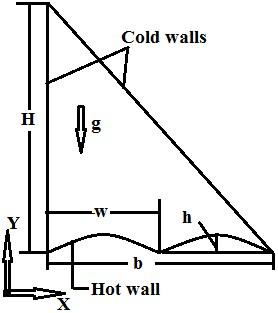Figure 1. Schematic diagram of problem geometry with coordinate system

Table 1. Thermo-physical properties of nanoparticles and water at Pr=6.2 [21, 22]

 Physical Properties Cp (j/kg k) ρ (kg/m3) k (W/m-k) β×10-5  (1/k) TiO2 686.2 4250 8.9538 0.9 CuO 540 6500 18 0.85 Al2O3 765 3970 40 0.85 Water 4179 997.1 0.613 21
2.1 Mathematical formulation

There are some specific presumptions are formed to prompt the governing equations of the nanofluid. Each wall of the cavity is presumed as impervious. Heat transfer by radiation is efficaciously negligible among the walls. The flow is assumed Newtonian, incompressible and steady. The nanoparticles and base fluid are presumed thermodynamically balance, no slip occurs between them and both of them are supposed to flow with the same velocity. The variation in the thermal properties of the fluid is assumed unchanged excluding the density variation in the y-direction (Boussinesq approximation). Under these assumptions, the two-dimensional mass, momentum, and energy equations are expressed as :

$\frac{\partial u}{\partial x}+\frac{\partial v}{\partial y}=0$  (1)

$u \frac{\partial u}{\partial x}+v \frac{\partial u}{\partial y}=-\frac{1}{\rho_{n f}} \frac{\partial p}{\partial x}+v_{n f}\left(\frac{\partial^{2} u}{\partial^{2} x}+\frac{\partial^{2} u}{\partial^{2} y}\right)$  (2)

$u \frac{\partial v}{\partial x}+v \frac{\partial v}{\partial y}=-\frac{1}{\rho_{n f}} \frac{\partial p}{\partial y}+v_{n f}\left(\frac{\partial^{2} v}{\partial^{2} x}+\frac{\partial^{2} v}{\partial^{2} y}\right)+\frac{(\rho \beta)_{n f}}{\rho_{n f}} g\left(T-T_{c}\right)$ (3)

$u \frac{\partial T}{\partial x}+v \frac{\partial T}{\partial y}=\alpha_{n f}\left(\frac{\partial^{2} T}{\partial^{2} x}+\frac{\partial^{2} T}{\partial^{2} y}\right)$   (4)

where αnf=knf/(ρcp)nf

The relative dimensionless parameters and numbers are:

$\mathrm{X}=\frac{x}{H}, \mathrm{Y}=\frac{y}{H}, \theta=\frac{T-T_{c}}{T_{h}-T_{c}}, \mathrm{Ra}=\frac{g \beta_{f}\left(T_{h}-T_{c}\right) H^{3}}{\alpha_{f} \vartheta}$  (5)

The variation in the effective density of the nanofluid at defined Prandtl number and reference temperature can be calculated by:

$\rho_{n f}=(1-\varphi) \rho_{f}+\varphi \rho_{n p}$ (6)

in this φ, ρnp, ρnf and ρf are denoted as nanoparticles volume fraction, the density of nanoparticles, the density of nanofluids density and density of the base fluid, respectively.

Correspondingly, the coefficient of thermal expansion and heat capacitance can be analyzed by:

$(\rho \beta)_{n f}=(1-\varphi)(\rho \beta)_{f}+\varphi(\rho \beta)_{n p}$  (7)

$\left(\rho C_{p}\right)_{n f}=(1-\varphi)\left(\rho C_{p}\right)_{f}+\varphi\left(\rho C_{p}\right)_{n p}$  (8)

The effectual thermal conductivity of the nanofluid is calculated through the model proposed by the Patel et al. :

$\frac{k_{n f}}{k_{f}}=1+\frac{k_{n p} A_{n p}}{k_{f} A_{f}}+c P e \frac{k_{n p} A_{n p}}{k_{f} A_{f}}$    (9)

where kf is the coefficient of thermal conductivity of the pure fluid, knp is the thermal conductivity of the dispersed nanoparticles and c is the experimental constant which value is to be c=25000. The Peclet number (Pe) and Anp/Af are defined as:

$P e=\frac{u_{b} d_{n p}}{\alpha_{f}}, \frac{A_{n p}}{A_{f}}=\frac{d_{n p}}{d_{f}} \frac{\varphi}{1-\varphi}$  (10)

ub is the Brownian motion of the particles which is described by:

$u_{b}=\frac{2 T k_{b}}{\pi \mu_{f} d_{n p}^{2}}$    (11)

where kb is the Boltzman Constant (kb=1.3807x10-23J/K). dnp is the diameter of solid particles which taken as (dnp=38 nm) and df is the size of molecule of the base fluid (df =2$\dot{A}$) .

The nanofluid effective viscosity is determined by Brinkman model  as below,

$\mu_{n f}=\frac{\mu_{f}}{(1-\varphi)^{2.5}}$  (12)

The dimensional boundary condition which is used to solve the governing equations is as follows:

T=Th, u=v=0 at y=0 and 0<x<b for base hot wall

T=Tc, u=v=0 at x=0 and 0<y<H for side cold wall (13)

T=Tc, u=v=0 at 0<x<b and 0<y<H for inclined cold wall

3. Numerical Techniques

Finite volume scheme is accompanied to solve the presented governing equations for the associated boundary conditions which are available in commercially obtainable software, FLUENT 6.3 . SIMPLE algorithm and second order upwind technique are involved for pressure-velocity coupling and to discretize the momentum and energy equations. The locally varying heat transfer on the base hot wall can be calculated by local Nusselt number:

$N u_{x}=-\frac{k_{n f}}{k_{f}}\left(\frac{\partial T}{\partial y}\right)_{y=0}$  (14)

where $\frac{k_{n f}}{k_{f}}$ is resolute using equation (8). However, the average Nusselt number is defined by:

$N u=\int_{0}^{b} N u_{x} d X$   (15)

The Nu (average) is determined from amended the Nusselt number which is recommended by Yesiloz and Aydin  to prevail on the singularity issue in a triangular enclosure:

$N u=\left(\int_{0}^{b} N u_{x} d x\right) \frac{\pi}{2}$    (16)

Relatively, the stream function is demarcated as:

$u=\frac{\partial \psi}{\partial y}, v=-\frac{\partial \psi}{\partial x}$  (17)

and the dimensionless stream function which is determined from:

Ѱ = ψ/α  (18)

3.1 Grid independence check and code validation

The wedge primitive grid shown in Figure 2 has been chosen for the study as this grid system is recommended by Triveni and Panua  and yesiloz and Aydin .  However, the grid system of 120 x 120 has been adopted for the present problem as Triveni and Panua  conducted the study at this grid size which is shown in Table 2.

## figure_2.jpgFigure 2. Suggested wedge primitive grid

Table 2. Result of grid independency test

 No. of grids in X-Y Ψmax Deviation (%) Nu Deviation (%) 60×60 36.04 14.95 80×80 34.62 3.94 15.01 0.40 100×100 33.05 4.53 15.02 0.07 120x120 31.90 3.50 15.05 0.19

The present study is conducted for water filled right triangular cavity retaining the similar boundary conditions at Ra=105 and Ra=5×105. The results are validated qualitatively by the available experimental and numerical outcomes of Yesiloz and Aydin  as shown in Figures 4 & 5. All the results are validated quantitatively shown in Figures 3, and 4 which unveiled relatively decent matching along with the published results.

## figure_3a1.jpg## figure_3a2.jpgѰmax= 12.094, Ѱmin=0

## figure_3b1.jpg## figure_3b2.jpgѰmax=25.47, Ѱmin=0

Figure 3. Experimental and Numerical streamlines (left and middle) from Yesiloz and Aydin and numerical simulation (right) from the present study for a) Ra=105 and b) Ra=5×105

## figure_4a1.jpg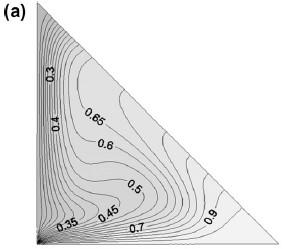## figure_4a2.jpg## figure_4b1.jpg## figure_4b2.jpgFigure 4. Experimental and Numerical isotherms (left column) from Yesiloz and Aydin and numerical isotherms (right column) from the present study for a) Ra=105 and b) Ra=5×105
4. Outcomes and Deliberations

The analysis of natural convection problem is executed within the right-triangular cavity for various types of nanoparticles (TiO2, CuO, and Al2O3) mixed with base fluids, a wide range of concentration of nanoparticles (0.01=φ=0.04) and Rayleigh number (105=Ra=107).  The flow of fluid is pesented by streamlines and the isotherm contours show the heat flow while the rate of transfer of heat is quantitatively shown by means of Nusselt number (local and average).

4.1 The effect of concentration of nanoparticles

Figure 5 encapsulates the consequences of volume fractions in the fluid flow and temperature distributions at Ra=1x106. It is noted from the figures that three vortexes of streamlines are shaped within the cavity for all the volume fractions and out of them the bigger vortex is rotated in counterclockwise while smaller vortexes in a clockwise direction. The inner cell of the bigger vortex is elliptic and widened in the case of pure fluid but once the nanoparticles are added to the base fluid, the width of the bigger cell is getting reduced. Similarly, the upper vortex is having two cells in case of pure fluid; the interior cell is becoming smaller and smaller with the intensification of volume fractions and finally, the cell vanishes at φ=0.04. It happens because the viscosity of nanofluid is raised with the increment of nanoparticles concentrations into the base fluid. Nevertheless, the thermal conductivity of the nanofluid is increased with the increase of volume fractions of nanoparticles and therefore, the global heat transfer rate of the cavity is mounted. It is worthy to mention that the increase in viscosity does not signify the full domination of conduction over convection.

φ = 0.00

## figure_511.jpg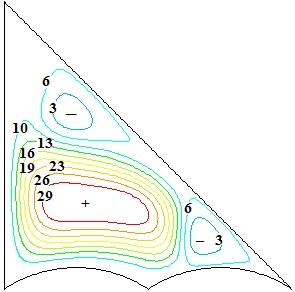Ψmax = 32.4

## figure_512.jpgφ = 0.01

## figure_521.jpgΨmax = 33.4

## figure_522.jpg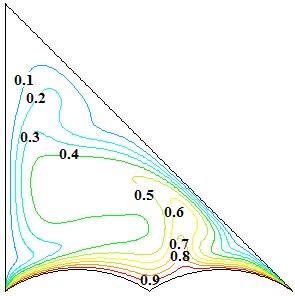φ = 0.02

## figure_531.jpgΨmax = 34.4

## figure_532.jpgφ = 0.03

## figure_541.jpgΨmax = 35.3

## figure_542.jpg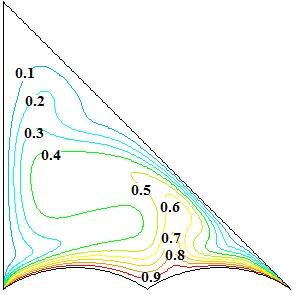φ = 0.04

## figure_551.jpgΨmax = 36.3

## figure_552.jpg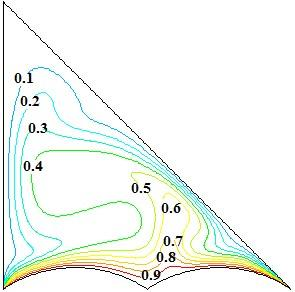Figure 5. Streamlines (left) and isotherms (right) for different volume fractions of Al2O3 at Ra =1x106

## figure_6.jpgFigure 6. Variation in convection coefficient with nanoparticles concentration of Al2O3 at Ra=106

The convection current of the nanofluid is also increased along with conduction with the increase of nanoparticles concentration as shown in Figure 6 and hence, the stream function of the nanofluid is more than the base fluid. The higher magnitude of stream function (Ψmax=36.3) is found for volume fractions φ=0.04 compare to the stream function (Ψmax=32.4) of base fluid. Figure 5 shows the variation in the isotherms for different concentration of nanoparticles on the right side. The isotherms are upraised adjacent to the hot wall and concentrated more near the inclined cold wall rather than the side cold wall. It is happening due to the inclination of the wall of the cavity which brings the isothermal walls in closer contact in contrast to the side cold wall. It is remarkable to mention that any variation in the isotherms rarely occurs with the increase of volume fractions.

## figure_7.jpgFigure 7. Variation of local Nusselt number with distance for different nanoparticle concentration of Al2O3 at Ra=1x106

Figure 7 displays the variation of local Nusselt number against the bottom wall of the enclosure filled with Al2O3–water nanofluid at Ra = 1x106. It is observed from the figure that the local Nusselt number is improved with the increase of the nanoparticles concentrations. The increment in the local Nusselt number at the left side of the cavity is according to the shape of the bottom wall but a titanic increase is found at the right side. The peak value of the LNN is calculated 644. 5, 617.1, 591.5, 565.9 and 541.8 at the right side edge of the cavity for φ=0.04, 0.03, 0.02, 0.01 and 0.00 respectively. This emphasized that the LNN is enhanced with the escalation of nanoparticles concentrations and this phenomenon is remarkably substantial nearby the right side of the triangular enclosure.

## figure_8.jpgFigure 8. Variation in average Nusselt number variation with volume fraction for different types of nanofluid at Ra=1x106

The variation of average Nusselt number is plotted against the volume fractions for different nanofluids at Rayleigh number 106 which is shown in Figure 8. From the plot, it is observed that the rate of heat transfer is significantly enhanced with the increment of volume fractions of the nanoparticles. However, the percentage variation in average Nusselt can be seen from the Table 3 and 4 which depicts the effect of different types of nanofluids and impact of different concentrations of nanoparticles. It becomes prominently clear that the Al2O3–water nanofluid plays a momentous role in heat transfer compared to CuO and TiO2–water nanofluid.

Table 3. Percentage variation of average Nusselt number for different types of nanofluids

 Average Nusselt number Ra = 1x106 TiO2 CuO Al2O3 φ = 0.01 35.47 35.71 36.60 φ = 0.00 35.20 35.20 35.20 Difference (%) 0.8% 1.5% 4% φ = 0.02 35.71 36.45 38.04 φ = 0.00 35.20 35.20 35.20 Difference (%) 1.5% 3.6% 8.1% φ = 0.03 35.96 37.00 39.45 φ = 0.00 35.20 35.20 35.20 Difference (%) 2.2% 5.1% 12.1% φ = 0.04 36.30 37.64 40.95 φ = 0.00 35.20 35.20 35.20 Difference (%) 3.1% 7% 16.3%

Table 4. Percentage variation of average Nusselt number for different concentrations of nanoparticles
 Average Nusselt number Ra = 1x106 φ = 0.01 φ =0.02 φ = 0.03 φ = 0.04 CuO 35.71 36.45 37.00 37.64 TiO2 35.47 35.71 35.96 36.3 Difference (%) 0.7% 2.1% 2.9% 3.7% Al2O3 36.60 38.04 39.45 40.95 CuO 35.71 36.45 37.00 37.64 Difference (%) 2.5% 4.4% 6.6% 8.8% Al2O3 36.60 38.04 39.45 40.95 TiO2 35.47 35.71 35.96 36.3 Difference (%) 3.2% 6.5% 9.7% 12.8%

4.2 The effects of Rayleigh number

This section, shown in Figure 9, exhibits the deviation of streamlines and isotherms for volume fractions φ=0.02 of CuO at different Rayleigh number. It can be observed from the streamlines that two vortexes are formed inside the enclosure for first two Rayleigh number. The left cell is rotated in anticlockwise while the right cell in a clockwise direction. The flow rotation for the left vortex is higher than the right side vortex. It occurs due to the constriction between the hot and cold wall which restricts the flow motion of the fluid at the nook while this phenomenon is not befallen at the left side of the cavity. The convection current is not strong enough at low Rayleigh number since the buoyancy force is weak. But, as the Rayleigh number is increased, the fluid particles possess high heat energy from the hot wall which makes the buoyancy force stronger. Hence, the flow patterns are changed considerably with the increase of Rayleigh number. In the last three Rayleigh number, the streamlines get fragmented into three vortexes, and the value of stream function is increased accordingly. The extreme value of stream function (Ψmax=75.6) is found at higher Rayleigh number while at lower Ra, the stream function is calculated Ψmax=21.7. The corresponding isotherms are moved away from each other at lower Rayleigh number. These are not in fully touched with the cold walls and situated far away from the upper corner of the enclosure. The isotherms are analogous to each other near the hot wall, come closer to each other and grow up towards the upper edge of the triangular cavity with the increase of Rayleigh number. The isotherms with θ<0.6 are getting clustered near the cold walls as the Rayleigh number is increased, while, the isotherms with θ>0.6 are stick to the hot wall at higher Rayleigh number. This explicates that the fluid flow rate is being intensified along with the escalation of Rayleigh number and hence the rate of heat transfer is uplifted.

Figure 10 illustrates the effect of Rayleigh number on the local Nusselt number for φ=0.02 of CuO. The local Nusselt number is varied according to the shape of the hot wall with the variation of Rayleigh number. It is conspicuous to indicate that the local Nusselt number is observed maximum at the crest of the caterpillar curve for higher Rayleigh number. At the right side of the cavity, heat transfer by conduction is well prevailed since some fluid particles get stuck due to the compactness at the corner while beside the corner, heat transfer is occurred due to the convection. That is why the local heat transfer rate is highly pronounced at the right corner of the cavity. The extreme value of local Nusselt number is obtained (Nux=565.192) at Ra=1x107.

Ra = 1x105

## figure_911.jpgΨmax=21.7

## figure_912.jpgRa = 5x105

## figure_921.jpg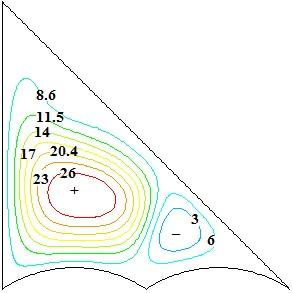Ψmax=28.7

## figure_922.jpgRa = 1x106

## figure_931.jpgΨmax=33.2

## figure_932.jpg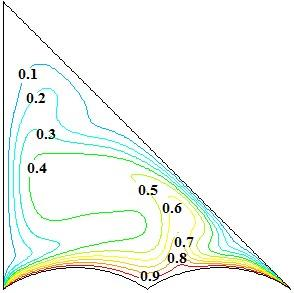Ra = 5x106

## figure_941.jpgΨmax=62.9

## figure_942.jpgRa = 1x107

## figure_951.jpgΨmax=75.6

## figure_952.jpg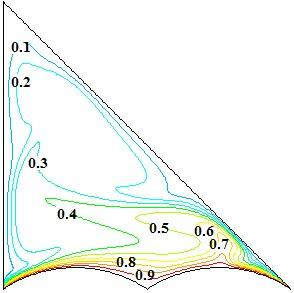Figure 9. Streamlines (left) and isotherms (right) for different Rayleigh number at φ=0.02 of CuO

## figure_10.jpg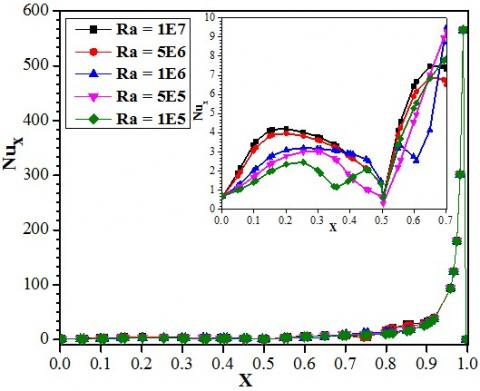Figure 10. Local Nusselt number along with hot wall for different Rayleigh number for φ=0.02 of CuO

Figure 11 (a, b and c) depicts the plot of Nu against Ra for various nanofluids. In the case of TiO2–water nanofluid, the heat transfer rate is increased with Ra but the rate of heat transfer is not augmented in considerable amount with the increase of the percentage of nanoparticles volume fractions. But, the considerable improvement in average Nusselt number is found for Al2O3-water nanofluid. The variation can also be observed from the Table 5-7. From the data, it is clear that the average Nusselt number is enhanced with the increase of Rayleigh number but the percentage increment in heat transfer rate is decreased with the increase of Rayleigh number.

## figure_11a.jpg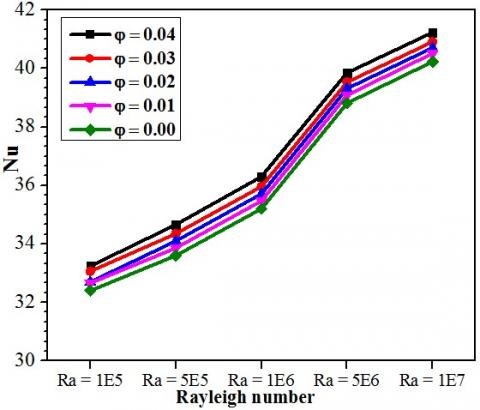(a)

## figure_11b.jpg(b)

## figure_11c.jpg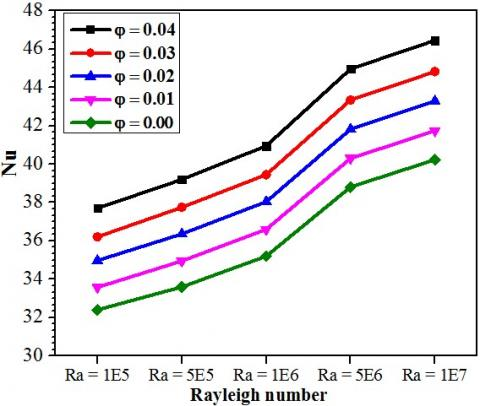(c)

Figure 11. Average Nusselt number with Rayleigh number for a) TiO2, b) CuO and c) Al2O3

Table 5. Percentage variation of average Nusselt number for TiO2-water nanofluids

 Average Nusselt number water TiO2 φ = 0.00 φ = 0.01 φ = 0.02 φ = 0.03 φ = 0.04 Ra = 5x105 33.60 33.86 34.10 34.35 34.66 Ra = 1x105 32.40 32.66 32.70 33.06 33.24 Difference (%) 3.7% 3.7% 4.3% 4% 4.3% Ra = 1x106 35.20 35.47 35.71 35.96 36.30 Ra = 1x105 32.40 32.66 32.70 33.06 33.24 Difference (%) 8.6% 8.6% 9.2% 8.8% 9.2% Ra = 5x106 38.81 39.1 39.31 39.53 39.85 Ra = 1x105 32.40 32.66 32.70 33.06 33.24 Difference (%) 19.8% 19.7% 20.2% 19.6% 20% Ra = 1x107 40.23 40.51 40.71 40.91 41.23 Ra = 1x105 32.40 32.66 32.70 33.06 33.24 Difference (%) 24.2% 24% 24.5% 23.7% 24%

Table 6. Percentage variation of average Nusselt number for CuO-water nanofluids
 Average Nusselt number water CuO φ = 0.00 φ = 0.01 φ = 0.02 φ = 0.03 φ = 0.04 Ra = 5x105 33.60 34.07 34.82 35.36 36.10 Ra = 1x105 32.40 32.84 33.46 34.01 34.72 Difference (%) 3.7% 3.7% 4% 4% 4% Ra = 1x106 35.20 35.71 36.45 37.00 37.64 Ra = 1x105 32.40 32.84 33.46 34.01 34.72 Difference (%) 8.6% 8.7% 9% 8.8% 8.4% Ra = 5x106 38.81 39.38 40.12 40.70 41.37 Ra = 1x105 32.40 32.84 33.46 34.01 34.72 Difference (%) 19.8% 19.9% 19.9% 19.7% 19.2% Ra = 1x107 40.23 40.81 41.56 42.12 42.80 Ra = 1x105 32.40 32.84 33.46 34.01 34.72 Difference (%) 24.2% 24.3% 24.2% 23.8% 23.3%

Table 7. Percentage variation of average Nusselt number for Al2O3-water nanofluids
 Average Nusselt number water Al2O3 φ = 0.00 φ = 0.01 φ = 0.02 φ = 0.03 φ = 0.04 Ra = 5x105 33.60 34.95 36.36 37.75 39.20 Ra = 1x105 32.40 33.58 34.97 36.21 37.70 Difference (%) 3.7% 4% 4% 4.3% 4% Ra = 1x106 35.20 36.60 38.04 39.45 40.95 Ra = 1x105 32.40 33.58 34.97 36.21 37.70 Difference (%) 8.6% 9% 8.8% 8.9% 8.6% Ra = 5x106 38.81 40.30 41.82 43.34 44.96 Ra = 1x105 32.40 33.58 34.97 36.21 37.70 Difference (%) 19.8% 20% 19.6% 19.7% 19.3% Ra = 1x107 40.23 41.74 43.28 44.82 46.45 Ra = 1x105 32.40 33.58 34.97 36.21 37.70 Difference (%) 24.2% 24.3% 23.8% 23.8% 23.2%
5. Conclusions

A detailed study of free convection has been conducted numerically in caterpillar shape right triangular cavity filled with pure fluid water and different type nanofluids. The aspect ratio (d) of the caterpillar curvature is maintained at 0.15 for the present study. Three different types of nanoparticles such as TiO2, CuO, and Al2O3, the different volume fraction of the nanoparticles and different Raleigh number havebeen used to emphasize the effect of the parametric study.

• The fluid flow is diminutively changed with the variation of the volume fraction of the nanoparticles.
• The fluid flow is followed the same flow pattern for all three nanofluids at fixed Rayleigh number.
• The augmentation in the rate of heat transfer occurs with the increment of volume fraction for all nanofluids.
• The Nu is obtained higher for Al2O3–water nanofluid followed by CuO and TiO2 respectively. The percentage rate of heat transfer is found very less for TiO2–water nanofluid.
• The rate of heat transfer for nanofluids is very much significant at a lower value of Rayleigh number than the higher Rayleigh number.
• The heat transfer rate is enhanced for all Rayleigh number.
Nomenclature
 g acceleration due to gravity in m/s2 H vertical length in m b horizontal length in m s inclination extent in m h apex of the caterpillar curve in m w breadth of the curvature in m n perpendicular direction to the plane A area in m2 d diameter in m p pressure in N/m2 u,v velocity components in m/s ub Brownian motion velocity in m/s x,y Cartesian co-ordinates system T temperature in K Th temperature of hot wall in K Tc temperature of cold wall in K Pr Prandtl number Gr Grashof number Pe Peclet number Nux Nusselt number (Local) Nu Nusselt number (Average) Greek symbols α thermal diffusivity inm2/s β coefficient of thermal expansion in 1/K k conduction coefficient in w/mK ρ density in Kg/m3 µ dynamic viscosity inN-s/m2 υ kinematic viscosity inm2/s ψ stream function in Kg/s δ curvature aspect ratio (h/w) Ψ dimensionless stream function Subscripts h hot wall c cold wall f basefluid np nanoparticle nf nanofluid
References

 Ben, N.K., Chouikh, R., Kerkeni, C., Guizani, A. (2006). Numerical study of the natural convection in cavity heated from the lower corner and cooled from the ceiling. Applied Thermal Engineering, 26: 772–775. https://doi.org/10.1016/j.applthermaleng.2005.09.011

 Triveni, M.K., Panua, R.S. (2018). Study of free convection in a double heating strips triangular cavity for various arrangements of cold walls. Arabian Journal for Science and Engineering, 43(3): 1455-1473. https://doi.org/10.1007/s13369 017-2977-1

 Basak, T., Aravind, G., Roy, S. (2009). Visualization of heat flow due to natural convection within triangular cavities using Bejan’S heatline concept. International Journal of Heat Mass Transfer, 52: 2824–2833. https://doi.org/10.1016/j.ijheatmasstransfer.2008.10.034

 Triveni, M.K., Panua, R.S. (2017). Numerical analysis of natural convection in a triangular cavity with different configuration of hot wall. International Journal of Heat and Technology, 35: 11-18. https://doi.org/10.18280/ijht.350102

 Morsli, S., Sabeur-Bendehina, A. (2013). Entropy generation and natural convection in square cavities with wavy walls. Journal of Applied Mechanics and Technical Physics, 54: 913–920. https://doi.org/10.1134/S0021894413060060

 Bhavnani, S.H., Bergles, A.E. (1991). Natural convection heat transfer from sinusoidal wavy surface. Warme-und Stoffubertragung, 26: 341-349. https://doi.org/10.1007/BF01591667

 Rahman, M.M., Öztop, H.F., Ahsan, A., Orfi, J. (2012). Natural convection effects on heat and mass transfer in a curvilinear triangular cavity. International Journal of Heat and Mass Transfer, 55: 6250–6259. https://doi.org/10.1016/j.ijheatmasstransfer.2012.06.055

 Rahman, M.M., Saidur, R., Mekhilef, S., Uddin, M.B., Ahsan, A. (2013). Double-diffusive buoyancy induced flow in a triangular cavity with corrugated bottom wall: Effects of geometrical parameters. International Communication in Heat Mass Transfer, 45: 64–74. https://doi.org/10.1016/j.icheatmasstransfer.2013.04.002

 Choi, S.U.S. (1995). Enhancing thermal conductivity of fluids with nanoparticles. ASME Fluids Engineering Division, 231: 99–105.

 Zoubida, H., Oztop, H.F., Abu-Nada, E., Mataoui, A. (2012). A review on natural convective heat transfer of nanofluids. Renewable Sustainable Energy Reviews, 16: 5363–5378. https://doi.org/10.1016/j.rser.2012.04.003

 Esmaeilpour, M., Abdollahzadeh, M. (2012). Free convection and entropy generation of nanoflid inside an enclosure with different patterns of vertical wavy walls. International Journal of Thermal Sciences, 52: 127–136. https://doi.org/10.1016/j.ijthermalsci.2011.08.019

 Sheremet, M.A., Pop, I., Shenoy, A. (2016). Natural convection in a wavy open porous cavity filled with a nanofluid: Tiwari and Das’ nanofluid model. The European Physical Journal Plus, 131: 62. https://doi.org/10.1140/epjp/i2016-16062-2

 Abu-Nada, E., Oztop, H.F. (2011). Numerical analysis of Al2O3/water nanofluids natural convection in a wavy walled cavity. Numerical Heat Transfer Part A, 59: 403–419. https://doi.org/10.1080/10407782.2011.552363

 Sheikholeslami, M., Gorji-Bandpy, M., Ganji, D.D., Soleimani, S. (2014). Numerical convection heat transfer in a cavity with sinusoidal wall filed with CuO-water nanofluid in presence of magnetic field. Journal of Taiwan Institute of Chemical Engineering, 45: 40–49. https://doi.org/10.1016/j.jtice.2013.04.019

 Cho, C.C., Chen, C.L., Chen, C.K. (2013). Natural convection heat transfer and entropy generation in wavy-wall enclosure containing water-based nanofluid. International Journal of Heat and Mass Transfer, 61: 749–758. https://doi.org/10.1016/j.ijheatmasstransfer.2013.02.044

 Ghasemi, B., Aminossadati, S.M. (2010) Brownian motion of nanoparticles in a triangular enclosure with natural convection. International Journal of Thermal Sciences, 49: 931–940. https://doi.org/10.1016/j.ijthermalsci.2009.12.017

 Sheikhzadeh, G.A., Qomi, M.E.,  Hajialigol, N., Fattahi, A. (2012). Numerical study of mixed convection flows in a lid-driven enclosure filled with nanofluid using variable properties. Results in Physics, 2: 5–13. https://doi.org/10.1016/j.rinp.2012.01.001

 Ghasemi, B., Aminossadati, S.M. (2010). Mixed convection in a lid-driven triangular enclosure filled with nanofluids. International Communication in Heat and Mass Transfer, 37: 1142–1148. https://doi.org/10.1016/j.icheatmasstransfer.2010.06.020

 Rahman, M.M., Mojumder, S., Saha, S., Mekhilef, S., Saidur, R. (2014). Augmentation of natural convection heat transfer in triangular shape solar collector by utilizing water based nanofluids having a corrugated bottom wall. International Communication in Heat and Mass Transfer, 50: 117–127. https://doi.org/10.1016/j.icheatmasstransfer.2013.10.008

 Sheikhzadeh, G.A., Qomi, M.E., Hajialigol, N., Fattahi, A. (2012). Numerical study of mixed convection flows in a lid-driven enclosure filled with nanofluid using variable properties. Results in Physics, 2: 5–13. https://doi.org/10.1016/j.rinp.2012.01.001

 Mahmoudi, A.H., Shahi, M., Raouf, A.H., Ghasemian, A. (2010). Numerical study of natural convection cooling of horizontal heat source mounted in a square cavity filled with nanofluid. International Communications in Heat and Mass Transfer, 37: 1135–1141. https://doi.org/10.1016/j.icheatmasstransfer.2010.06.005

 Moraveji, M.K., Hejazian, M. (2013). Natural convection in a rectangular enclosure containing an oval-shaped heat source and filled with Fe3O4-water nanofluid. International Communications in Heat and Mass Transfer, 44: 135–146. https://doi.org/10.1016/j.icheatmasstransfer.2013.03.011

 Sheikholeslami, M. (2017). CuO-water nanofluid free convection in a porous cavity considering Darcy law. The European Physical Journal Plus, 132: 55. https://doi.org/10.1140/epjp/i2017-11330-3

 Sheikholeslami, M., Shehzad, S.A. (2018). Simulation of water based nanofluid convective flow inside a porous enclosure via non-equilibrium model. International Journal of Heat and Mass Transfer, 120: 1200-1212. https://doi.org/10.1016/j.ijheatmasstransfer.2017.12.132

 Raju, C.S.K, Sandeep, N. (2017). Unsteady Casson nanofluid flow over a rotating cone in a rotating frame filled with ferrous nanoparticles: A numerical study. Journal of Magnetism and Magnetic Materials, 421: 216-224. https://doi.org/10.1016/j.jmmm.2016.08.013

 Reddy, J.V., Sugunamma, V., Sandeep, N. (2018). Simultaneous effects of radiation and variable heat source/sink on MHD flow of keosene Fe3O4 ferroliquid over a bidirectional stretched geometry. Journal of Nanofluids, 7: 748-754. https://doi.org/10.1166/jon.2018.1490

 Triveni, M.K., Panua, R. (2016). Numerical simulation of natural convection in a triangular enclosure with caterpillar (C)-curve shape hot wall. International Journal of Heat and Mass Transfer, 96: 535–547. https://doi.org/10.1016/j.ijheatmasstransfer.2016.02.002

 Patel, H.E., Sundararajan, T., Pradeep, T., Dasgupta, A., Dasgupta, N., Das, S.K. (2005). A micro convection model for thermal conductivity of nanofluids. Pramana—Journal of Physics, 65(5): 863–869. https://doi.org/10.1007/BF02704086

 Mahmoudi, A.H., Shahi, M., Raouf, A.H., Ghasemian, A. (2010). Numerical study of natural convection cooling of horizontal heat source mounted in a square cavity filled with nanofluid. International Communications in Heat and Mass Transfer, 37: 1135-1141. https://doi.org/10.1016/j.icheatmasstransfer.2010.06.005

 Brinkman, H.C. (1952). The viscosity of concentrated suspensions and solution. The Journal of Chemical Physics, 20: 571–581. https://doi.org/10.1063/1.1700493

 Fluent User's Guide, Release 6.3.26, Fluent Incorporated. Fluent Inc. 2006-09-20.

 Yesiloz, G., Aydin, O. (2013). Laminar natural convection in right-angled triangular enclosures heated and cooled on adjacent walls. International Journal of Heat and Mass Transfer, 60: 365-374. https://doi.org/10.1016/j.ijheatmasstransfer.2013.01.009